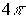A brief description of the commands is as follows.

• SGe_parameters: receives the list of the physical characteristics for the constituent elements of the desired N-soliton solution and generates the list of the Backlund parameters and initial positions of that elements in the form required by SGe_backlund command;
• SGe_backlund takes the list of the Backlund parameters and initial positions of the constituent elements and returns the \$N\$-soliton solution as a function of space and time variables;
• SGe_matrix calculates the solution at the nodes of the rectangular grid of space and time variables, eliminates the discontinuities multiple ofand returns the N-soliton solution in the matrix form;
• SGe_animate creates the 2D animation plots for the N-soliton solution obtained by SGe_matrix and SGe_backlund commands;
• SGe_pendulum calculates the solution by using the SGe_backlund command and creates an animation of the N-soliton solution in frame of the pendulum model;
• SGe_ribbon calculates the solution by using the SGe_backlund command and creates an animation of the N-soliton solution in frame of the elastic ribbon model.

A brief description of the parameters for the commands

The input data for the solution are as follows.

Title is the string which defines the title of the picture.

Parameter m defines the total number of basic elements. The one-soliton solutions, kink and antikink, and the two-soliton solutions, breather, kink-kink and kink-antikink solutions are considered as the basic elements.

Parameter v defines the physical characteristics for j-th basic element, 1 <= j <= m, in the following manner.

v[j, 1] is the type (charge) of the elements. It equals to +1 for kink, -1 for antikink, 0 for breather and for kink-antikink solutions and 2 for kink-kink solution;

v[j, 2] is the velocity of the basic element (this parameter can vary in the range from -1 to 1);

v[j, 3] is the frequency of breather, which can range from 0 to 1. This element should be equal to 0 for kink and antikink, 1 for kink-antikink solution and -1 for kink-kink solution;

v[j, 4] is the initial position of the element.

L0, L1 specify the range for space variable.

T0, T1 specify the range for time variable.

Nx and Nt specify the number of points of the space and time grids, respectively. For animated representations: Nx is the minimal number of points to be generated in the space range and Nt is the number of frames to be displayed.

Na is the number of arrows drawn in the picture.

Np is the number of pendulums drawn in the picture.